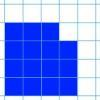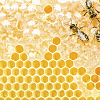Search by Topic

Resources tagged with Perimeter similar to Changing Areas, Changing Perimeters:

Filter by: Content type:
Age range:
Challenge level:

There are 14 results

Broad Topics > Measuring and calculating with units > PerimeterChanging Areas, Changing Perimeters

Age 11 to 14 Challenge Level:

How can you change the area of a shape but keep its perimeter the same? How can you change the perimeter but keep the area the same?Perimeter Challenge

Age 11 to 14 Challenge Level:

Can you deduce the perimeters of the shapes from the information given?Coins on a Plate

Age 11 to 14 Challenge Level:

Points A, B and C are the centres of three circles, each one of which touches the other two. Prove that the perimeter of the triangle ABC is equal to the diameter of the largest circle.Can They Be Equal?

Age 11 to 14 Challenge Level:

Can you find rectangles where the value of the area is the same as the value of the perimeter?Pick's Theorem

Age 14 to 16 Challenge Level:

Polygons drawn on square dotty paper have dots on their perimeter (p) and often internal (i) ones as well. Find a relationship between p, i and the area of the polygons.Smaller and Smaller

Age 7 to 14 Challenge Level:

Can you predict, without drawing, what the perimeter of the next shape in this pattern will be if we continue drawing them in the same way?Warmsnug Double Glazing

Age 14 to 16 Challenge Level:

How have "Warmsnug" arrived at the prices shown on their windows? Which window has been given an incorrect price?Perimeter Expressions

Age 11 to 14 Challenge Level:

Create some shapes by combining two or more rectangles. What can you say about the areas and perimeters of the shapes you can make?Perimeter Possibilities

Age 11 to 14 Challenge Level:

I'm thinking of a rectangle with an area of 24. What could its perimeter be?Some(?) of the Parts

Age 14 to 16 Challenge Level:

A circle touches the lines OA, OB and AB where OA and OB are perpendicular. Show that the diameter of the circle is equal to the perimeter of the triangleIs There a Theorem?

Age 11 to 14 Challenge Level:

Draw a square. A second square of the same size slides around the first always maintaining contact and keeping the same orientation. How far does the dot travel?On the Edge

Age 11 to 14 Challenge Level:

If you move the tiles around, can you make squares with different coloured edges?Coke Machine

Age 14 to 16 Challenge Level:

The coke machine in college takes 50 pence pieces. It also takes a certain foreign coin of traditional design...Contact

Age 14 to 16 Challenge Level:

A circular plate rolls in contact with the sides of a rectangular tray. How much of its circumference comes into contact with the sides of the tray when it rolls around one circuit?